Ex 7.3

Chapter 7 Class 10 Coordinate Geometry
Serial order wise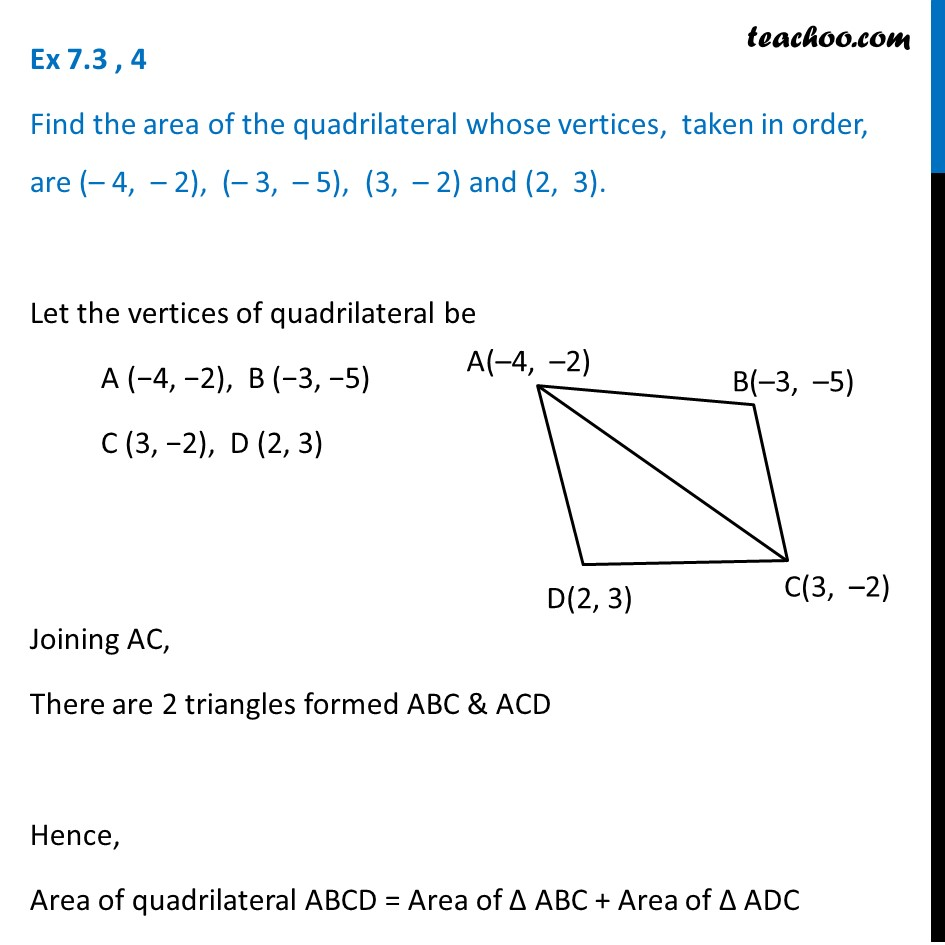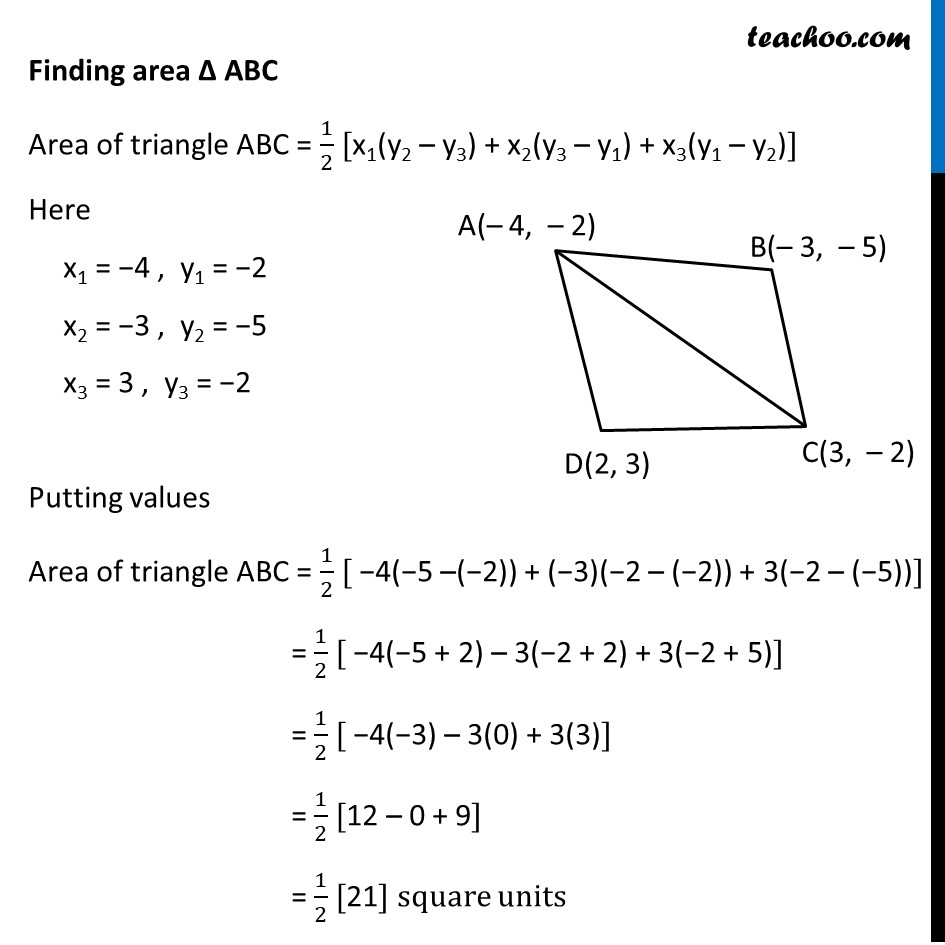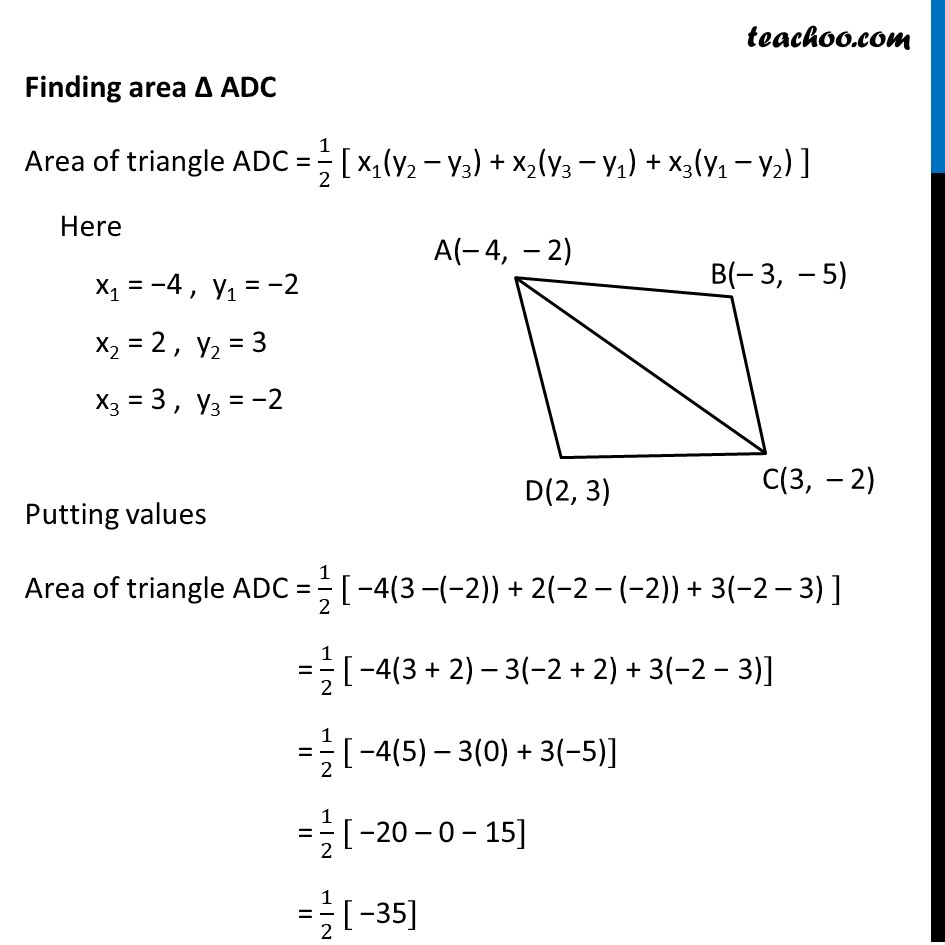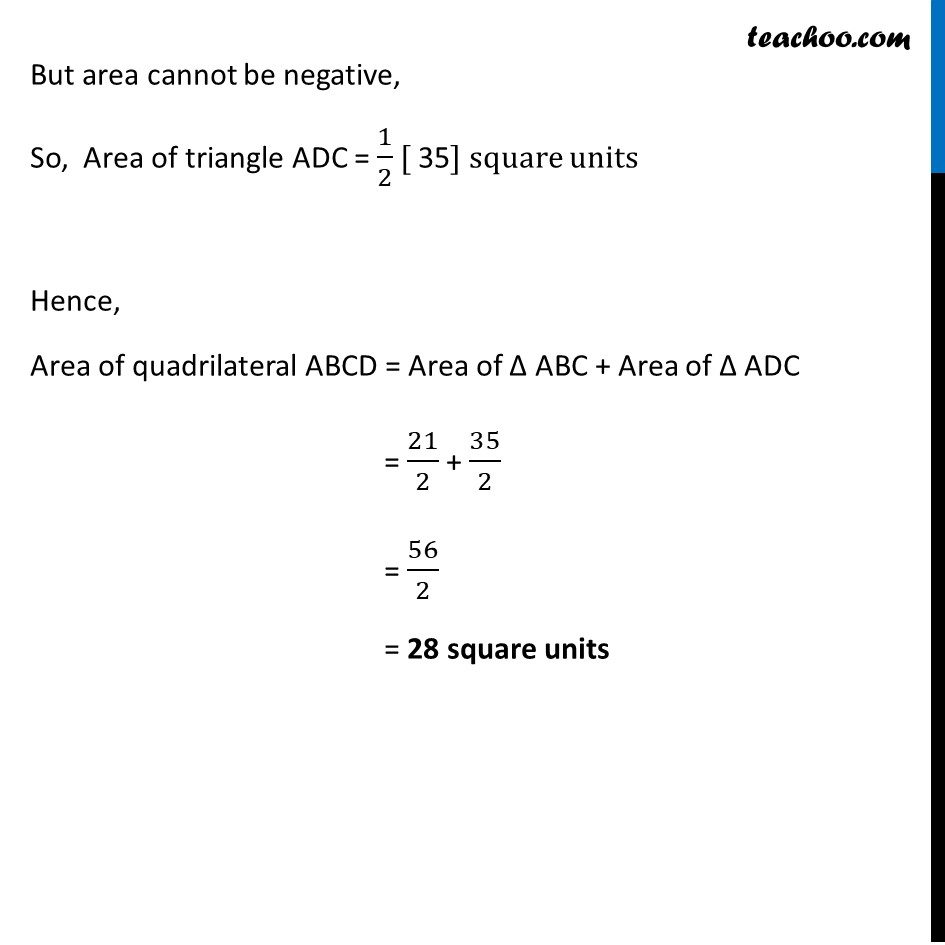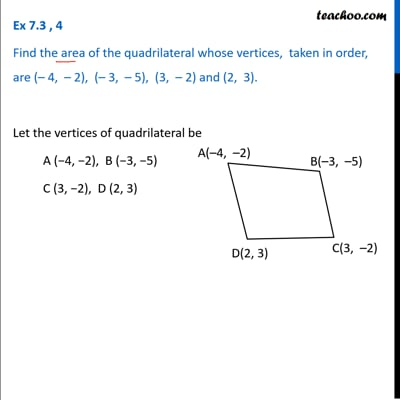This video is only available for Teachoo black users

### Transcript

Ex 7.3 , 4 Find the area of the quadrilateral whose vertices, taken in order, are (– 4, – 2), (– 3, – 5), (3, – 2) and (2, 3). Let the vertices of quadrilateral be A (−4, −2), B (−3, −5) C (3, −2), D (2, 3) Joining AC, There are 2 triangles formed ABC & ACD Hence, Area of quadrilateral ABCD = Area of ∆ ABC + Area of ∆ ADC Finding area ∆ ABC Area of triangle ABC = 1/2 [x1(y2 – y3) + x2(y3 – y1) + x3(y1 – y2)] Here x1 = −4 , y1 = −2 x2 = −3 , y2 = −5 x3 = 3 , y3 = −2 Putting values Area of triangle ABC = 1/2 [ −4(−5 –(−2)) + (−3)(−2 – (−2)) + 3(−2 – (−5))] = 1/2 [ −4(−5 + 2) – 3(−2 + 2) + 3(−2 + 5)] = 1/2 [ −4(−3) – 3(0) + 3(3)] = 1/2 [12 – 0 + 9] = 1/2  square units Finding area ∆ ADC Area of triangle ADC = 1/2 [ x1(y2 – y3) + x2(y3 – y1) + x3(y1 – y2) ] Here x1 = −4 , y1 = −2 x2 = 2 , y2 = 3 x3 = 3 , y3 = −2 Putting values Area of triangle ADC = 1/2 [ −4(3 –(−2)) + 2(−2 – (−2)) + 3(−2 – 3) ] = 1/2 [ −4(3 + 2) – 3(−2 + 2) + 3(−2 − 3)] = 1/2 [ −4(5) – 3(0) + 3(−5)] = 1/2 [ −20 – 0 − 15] = 1/2 [ −35] But area cannot be negative, So, Area of triangle ADC = 1/2 [ 35] square units Hence, Area of quadrilateral ABCD = Area of ∆ ABC + Area of ∆ ADC = 21/2 + 35/2 = 56/2 = 28 square units